# AI打LeetCode周赛进入前10%！秘诀：自然语言编程

AI书写代码还能这样玩

• 全程使用自然语言进行描述，通过一次沟通完成输出，因此下文所有发给Claude的指令均可以复现。
• 采用问题描述、解答思路、以及开头书写要求作为提问固定格式。
• 编程采用python代码输出。

# 用自然语言让AI打leetcode周赛

Leetcode第103场周赛题目1如下，k个元素的最大和：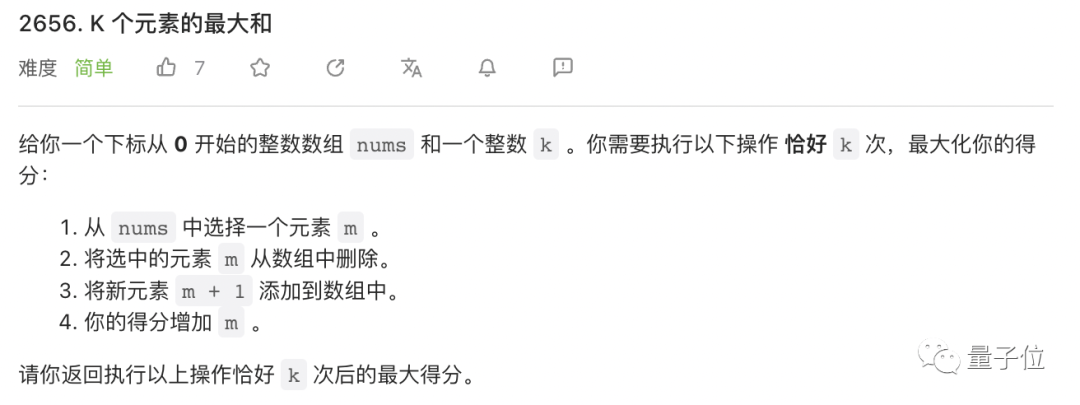``````问题描述如下：
'''

1.从 nums 中选择一个元素 m 。
2.将选中的元素 m 从数组中删除。
3.将新元素 m + 1 添加到数组中。
4.你的得分增加 m 。

'''

'''

'''

class Solution:
def maximizeSum(self, nums: List[int], k: int) -> int:``````

Claude回复内容如下：

``````这里是python代码实现:
python
class Solution:
def maximizeSum(self, nums: List[int], k: int) -> int:
m = max(nums)
return (2*m + k - 1) * k // 2

1. 首先找到数组中的最大值m。
2. 然后最大的得分公式为:m + (m+1) + (m+2) + ... + (m+k-1)
= (m + (m+k-1)) * k / 2
= (2*m + k - 1) * k / 2
3. 直接返回计算结果。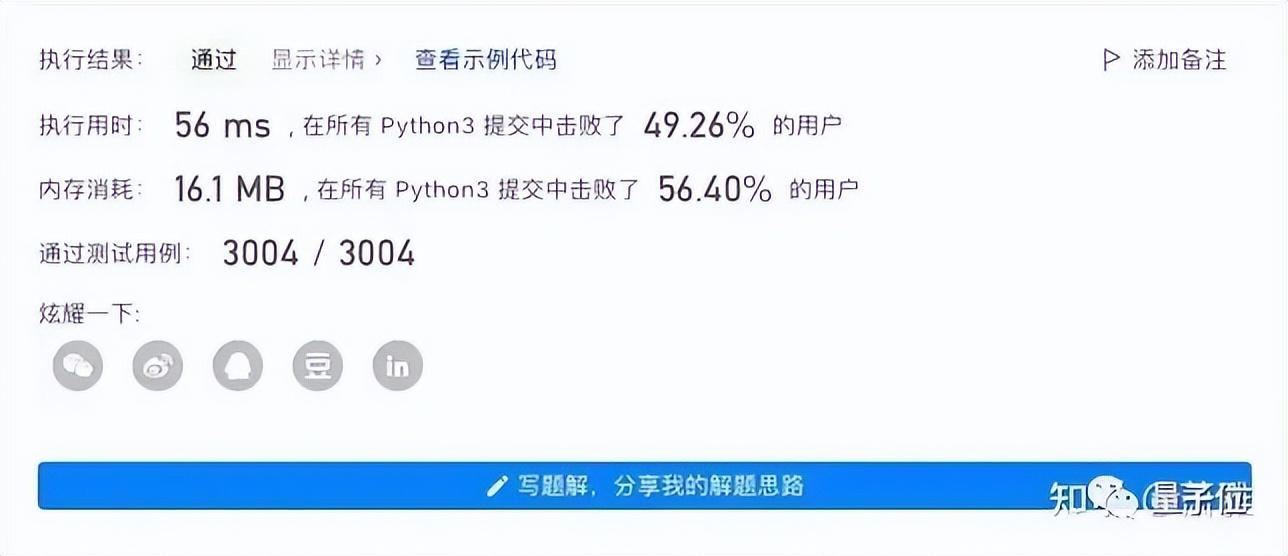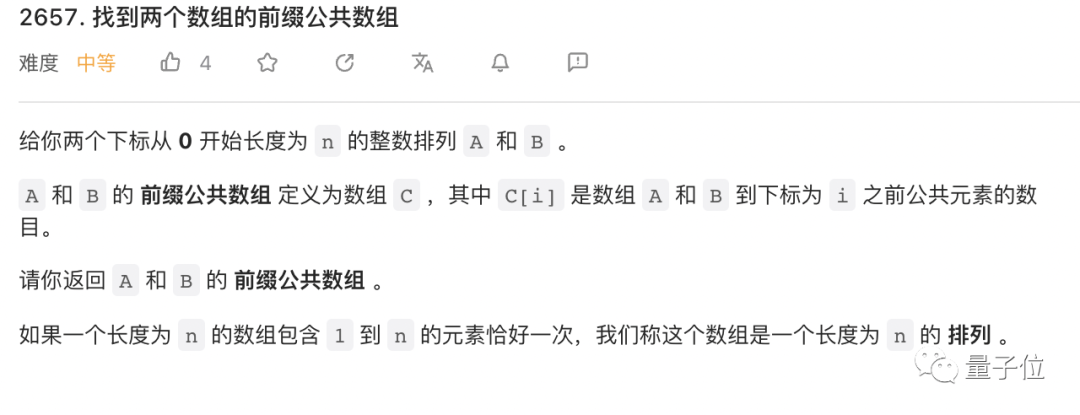``````问题描述如下：
'''

A 和 B 的 前缀公共数组 定义为数组 C ，其中 C[i] 是数组 A 和 B 到下标为 i 之前公共元素的数目。

'''

'''
1.存储公共前缀的大小
2.存储 a 和 b 中已经匹配过的元素
3.同时遍历 a 和 b 中的元素
4.将 a 中的当前元素 x 对应的二进制位置为 1，标记为已匹配
5.将 b 中的当前元素 y 对应的二进制位置为 1，标记为已匹配
6.计算当前公共前缀的大小，即 p 和 q 的二进制数中 1 的个数
7.返回公共前缀大小的列表
'''

class Solution:
def findThePrefixCommonArray(self, A: List[int], B: List[int]) -> List[int]:``````

Claude回复内容如下：

``````这里是优化后的python代码实现:
python
class Solution:
def findThePrefixCommonArray(self, A: List[int], B: List[int]) -> List[int]:
p, q = 0, 0
res = []
for i in range(min(len(A), len(B))):
p |= 1 << A[i]
q |= 1 << B[i]
res.append(bin(p&q).count('1'))
return res

1. p和q初始化为0,用来记录A和B数组中出现过的数字。
2. 同时遍历A和B数组的元素。
3. 通过p |= 1 << A[i]将A[i]位置1。
4. 通过q |= 1 << B[i]将B[i]位置1。
5. p & q得到两个数组公共元素,然后bin(p & q).count('1')计算1的个数,得到公共元素个数c。
6. 添加c到res。
7. 重复3-6直到A或B数组遍历结束。
8. 返回res。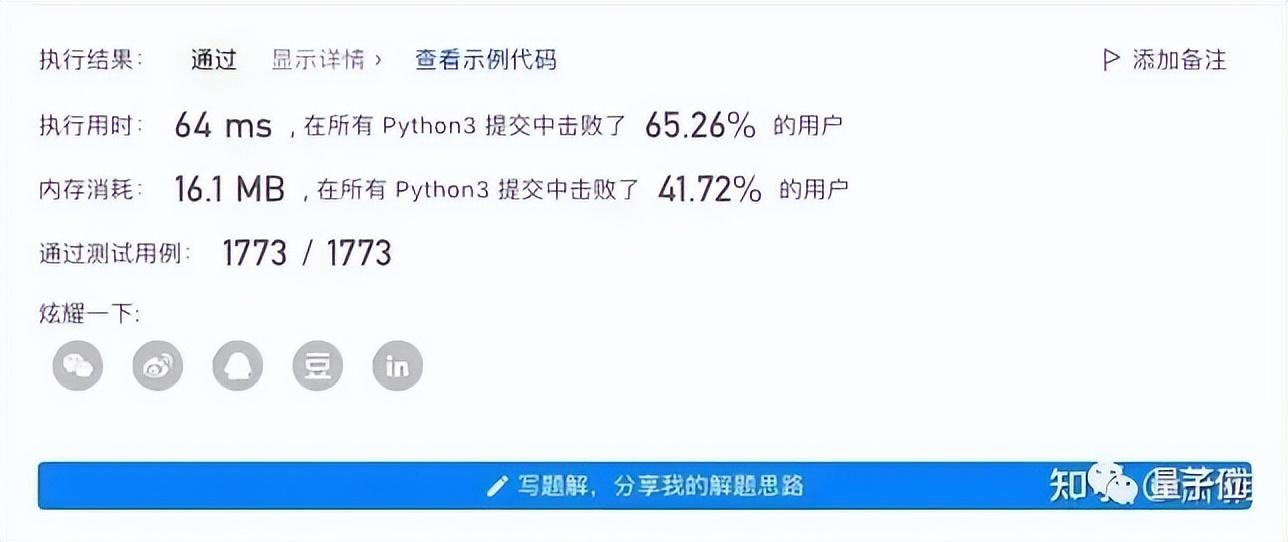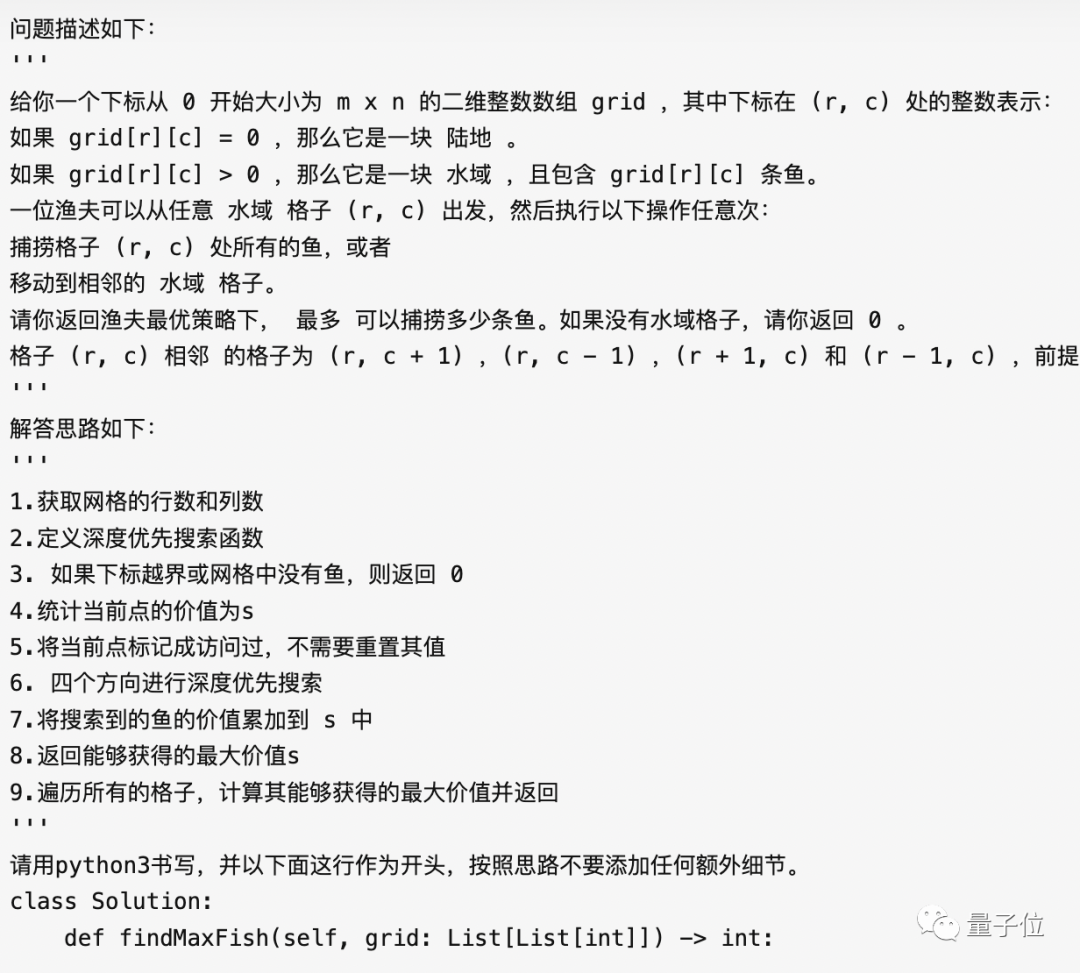``````问题描述如下：
'''

'''

'''
1.获取网格的行数和列数
2.定义深度优先搜索函数
3. 如果下标越界或网格中没有鱼，则返回 0
4.统计当前点的价值为s
5.将当前点标记成访问过，不需要重置其值
6. 四个方向进行深度优先搜索
7.将搜索到的鱼的价值累加到 s 中
8.返回能够获得的最大价值s
9.遍历所有的格子，计算其能够获得的最大价值并返回
'''

class Solution:
def findMaxFish(self, grid: List[List[int]]) -> int:``````

Claude回复内容如下：

``````这里是精简的python代码实现:
python
class Solution:
def findMaxFish(self, grid: List[List[int]]) -> int:
m, n = len(grid), len(grid)
def dfs(i, j):
if not (0 <= i < m and 0 <= j < n and grid[i][j] > 0): return 0
s = grid[i][j]
grid[i][j] = 0
s += dfs(i+1, j) + dfs(i-1, j) + dfs(i, j+1) + dfs(i, j-1)
return s
res = 0
for i in range(m):
for j in range(n):
res = max(res, dfs(i, j))
return res

1. 获取网格的行数m和列数n。
2. 定义dfs函数进行深度优先搜索。
3. 如果下标越界或网格中没有鱼,则返回 0。
4. 统计当前点grid[i][j]的鱼的数量s。
5. 将grid[i][j]标记为已访问,不需要重置其值 。
6. dfs搜索四个方向,并将搜索到的鱼的数量累加到s。
7. 返回s。
8. 遍历所有的格子,通过dfs计算其能够获得的最大价值,并更新res。
9. 返回res。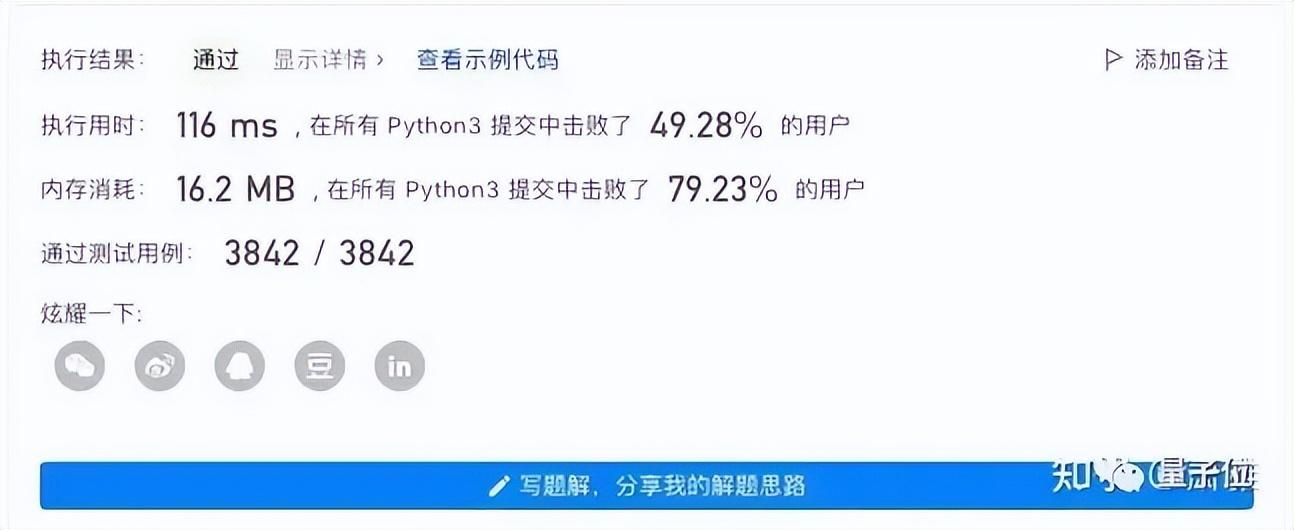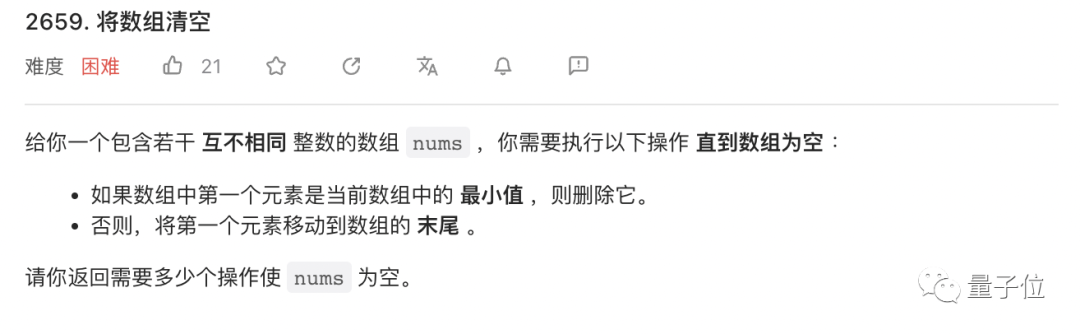``````问题描述如下：
'''

'''

'''

res用于存储清空数组所需的最少操作数，初始化为 0

'''

class Solution:
def countOperationsToEmptyArray(self, nums: List[int]) -> int:``````

Claude回复内容如下：

``````这里是python代码实现:
python
from sortedcontainers import SortedList

class Solution:
def countOperationsToEmptyArray(self, nums: List[int]) -> int:
n = len(nums)
heap = []
for i, num in enumerate(nums):
heappush(heap, (num, i))
pos = SortedList()
res = 0
li = -1
while heap:
num, i = heappop(heap)
if i > li:
d = pos.bisect_left(i) - pos.bisect_right(li)
res += i - li - d
else:
d = pos.bisect_right(n) - pos.bisect_right(li) + pos.bisect_left(i)
res += n - li + i - d
li = i
return res

1. 数组 nums 的长度为n。
2. 创建一个空的堆列表heap。
3. 对nums进行i,nums这个格式的遍历,再将当前元素值和对应索引组成后面格式的元组 (num, i) 加入到堆列表 heap 中,不要对这两个格式顺序做修改。
4. 用sortedcontainers里面的SortedList创建空的有序集合pos,用于存储已经删除的元素的下标。
5. res用于存储清空数组所需的最少操作数,初始化为0。
6. 哨兵li,上一个弹出的数的位置,初始化为-1。
7. 获取堆中的最小值,并计算清除该元素所需的步数,重复直到堆为空。
8. 如果i在上一个弹出元素li的后面,对于每个被弹出的元素,计算从i到li在pos中的有效元素数量d,即计算值在 li的右边且值小于 i 的元素数量。再将从当前位置到i之间的长度减去d,加入总步数res中。
9. 如果当前元素的位置在上一个被弹出元素li的前面,则计算从li到数组结尾的有效元素数量d,即集合中小于等于i的元素数量与集合中小于n的元素数量相加,再减去集合中小于li的元素数量为有效元素数量d。再将从当前位置li到数组末尾的元素数量加上i,再减去d,加入总步数res中。
10. 将元素i的下标加入已删除元素的有序集合pos中。
11. 更新上一个弹出的数位置li = i。
12. 返回清空数组所需的最少操作数res。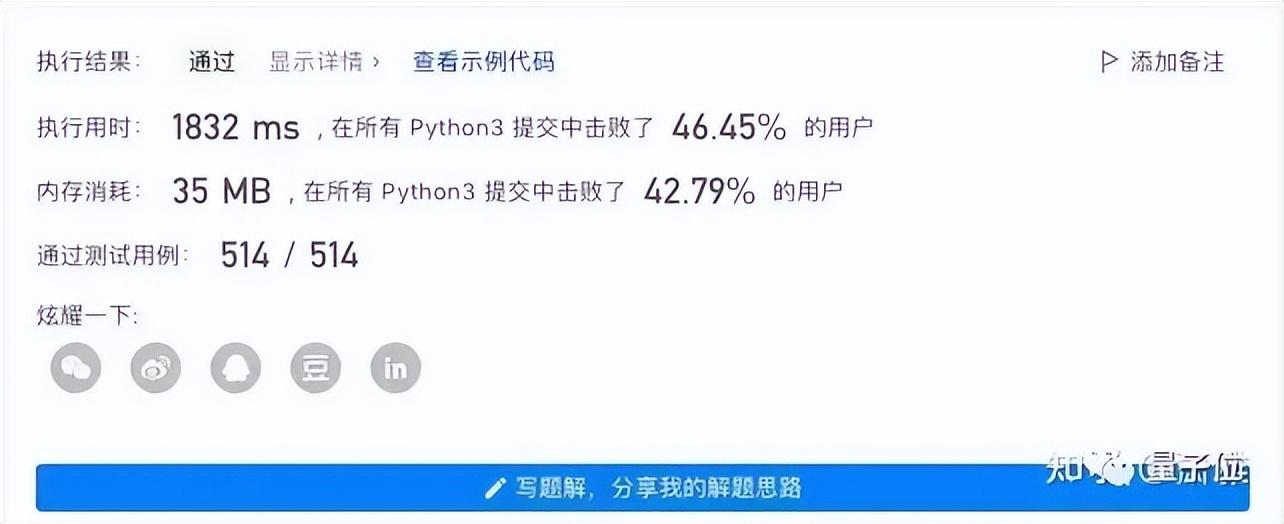# 实践后的思考

• 语言表述：用尽可能精准自然的语言来进行沟通回复，避免重复性词语，尽可能不使用可能/或许这种不确定性的描述。
• 语义理解：过于复杂或者专业的表达会增加理解难度，需要补充更为清晰易懂的技术背景，会得到更好的输出。
• 交互模式：用更为直接明了的命令形式进行表达，虽然不及聊天形式自然，但更为清晰简明。
• 规范结构：需要在表达方式和交互规则上增加一定框架，对输入输出都会有更为直观的展示。

# 能超过50%的周赛参与者

“比如说本身对c++非常熟悉，而可能这个代码需要python书写才能更匹配，哪怕对语言不是很了解，但是整体的思路和想法有很大共通的。”她补充解释道，“这个语言做的好的地方，相对的那个语言应该也会有对应的库，但是可以在不那么精通另一个语言的情况下依然很好的书写代码。”